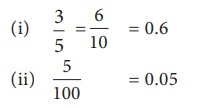Home | | Maths 7th Std | Fractions and Decimals

# Fractions and Decimals

Let us see the relationship between fractions and decimals. 1. Conversion of Fractions to Decimals 2. Conversion of Decimals to Fractions

Fractions and Decimals

Let us see the relationship between fractions and decimals.

1. Conversion of Fractions to Decimals

We are familiar with fraction as a part of a whole. The place value of the decimal digits of a number are tenths (1/10), hundredths (1/100) , thousandths (1/1000) and so on.

If the denominator of a fraction is any of 10,102 ,103, ... we can express them as decimals. Consider the example of distributing a box of 10 pencils to ten students. The portion of pencils given to 6 students will be 6/10 which can be expressed as 0.6.

If the denominator of a fraction is any number that can be made as powers of 10 using the concept of equivalent fractions, then it can also be expressed as decimals. Consider the example of sharing 5 peanut cakes among five friends. The share of one person is 1/5. To represent this fraction as a decinal number. We first convert the denominator into 10. This can be done by writing the equivalent fraction of  1/5, namely 2/10 . Now the decimal representation of 2/10 is 0.2

Think

Can you express the denominators of all fractions as powers of 10?

2. Conversion of Decimals to Fractions

As we convert fractions into a decimal number, the decimal numbers can also be expressed as fractions.

For example, let the price of brand ‘x’ slippers be 399.95

Expanding the above price, we get,

399.95 = 3 ×100 + 9 ×10 + 9 ×1 + 9 × 1/10 + 5×1/100

= 399 + 95/100 = 39995/100 = 7999/20

Similarly, if the price of brand ‘y’ slipper is ₹ 159.95, then it can be expressed in terms of fractions as below.

159.95 = 159 + 95/100 = 15995/100 = 3199/20

Try these

1. Convert the following fractions into the decimal numbers.

(i) 16/1000

(ii) 638/10

(iii) 1/20

(iv) 3/50

(i) 16/1000 = 0.016

(ii) 638 / 10 = 638

(iii) 1/20 × 5/5 = 5/100 = 0.05

(iv) 3/50 × 2/2 = 6/100 = 0.06

2. Write the fraction for each of the following:

(i) 6 hundreds + 3 tens + 3 ones + 6 hundredths + 3 thousandths.

(ii) 3 thousands + 3 hundreds + 4 tens + 9 ones + 6 tenths.

(i) 6 hudreds + 3 tens + 3 ones + 6 hundredths + 3 thousandths. . .

6 × 100 – 3 × 10 + 3 × 1+ 6 × 1/100 + 3 × 1/ 1000

600+ 30 + 3 + 6/100 + 3/1000

633063

(ii) 3 thousands + 3 hundreds + 4 tens + 9 ones + 6 tenths.

3 × 1000 + 3 ×  100 + 4 × 10 + 9 × 1+ 6 × 1/10

3000– 300 + 40 + 9 + 6/10

33496

3. Convert the following decimals into fractions.

(i) 0.0005

(ii) 6.24

(i) 0.0005 = 5 / 10000 = 1 / 2000

(ii) 6.24 = 624 / 100 = 156 / 25

Example 1.6

Write the shaded portion of the figures given below as a fraction and as a decimal number.SolutionExample 1.7

Express the following fractions as decimal numbers.SolutionExample 1.8

Write the following fractions as decimals.Solution

(i) We have to find a fraction equivalent to 2/5 whose denominator is 10.(ii) We have to find a fraction equivalent to 3/4 with denominator 100. Since there is no whole number that gives 10 when multiplied by 4, let us make the denominator as 100.(iii) In 9/1000 , tenth and hundredth place is zero.

Therefore, = 9/1000 = 0.009

(iv) We have to find a fraction equivalent to 1/50 whose denominator is 100.(v) In 3 1/5, keep the whole number 3 as such, we can find a fraction equivalent to 1/5 with denominator 10.Example 1.9

Convert the following into simplest fractions.

(i) 0.04

(ii) 3.46

(iii) 0.862

SolutionExample 1.10

Find the decimal form of the following fractions.Solution= 23.608 (since hundredths place is not there,  it is taken as '0')

Example 1.11

Write each of the following as decimals.

(i) Four hundred four and five hundredths

(ii) Two and twenty five thousandths.

Solution

(i) Four hundred four and five hundredths.

= 404 + 5/100

= 404 + [ 0 × 1/10 + 5 × 1/100 ] = 404.05

(ii) Two and twenty five thousandths= 2.025 [as there is no tenth we take it as 0 tenth]

Note

For any decimal number, number of zeroes in the denominator and  number of decimal digits are equal.

Example 1.12

Express the following as fractions (i) A capsule contains 0.85 mg of medicine. (ii) A juice container has 4.5 litres of mango juice.

SolutionA decimal is a fraction written in a special form. Decimal comes from the Latin word 'decimus' which means tenth. It comes from the root word 'decem'

Tags : Number System | Term 2 Chapter 1 | 7th Maths , 7th Maths : Term 2 Unit 1 : Number System
Study Material, Lecturing Notes, Assignment, Reference, Wiki description explanation, brief detail
7th Maths : Term 2 Unit 1 : Number System : Fractions and Decimals | Number System | Term 2 Chapter 1 | 7th Maths